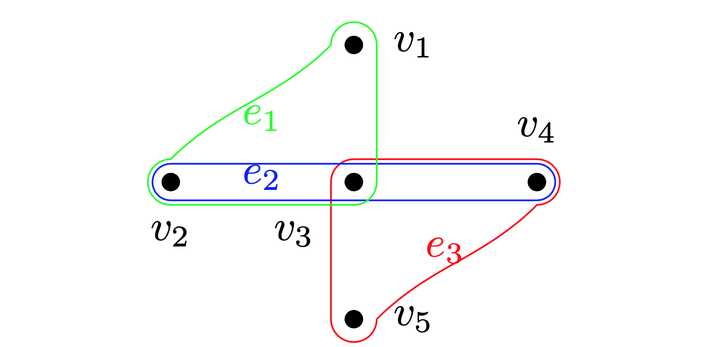# Hyperedge Prediction using Tensor Eigenvalue Decomposition### Abstract

Link prediction in graphs is studied by modeling the dyadic interactions among two nodes. The relationships can be more complex compared to simple dyadic interactions and could require the user to model super-dyadic association among nodes. Such interactions can be modeled using a hypergraph, which is a generalization of graph where a hyperedge can connect more than two nodes.
In this work, we consider the problem of hyperedge prediction in a k-uniform hypergraph. We utilize the tensor-based representation of hypergraphs and propose a novel interpretation of the tensor eigenvectors. This is further used to propose a hyperedge prediction algorithm. The proposed algorithm utilizes the Fiedler eigenvector computed using tensor eigenvalue decomposition of hypergraph Laplacian. The Fiedler eigenvector is used to evaluate the construction cost of new hyperedges, which is further utilized to determine the most probable hyperedges to be constructed. The functioning and efficacy of the proposed method are illustrated using some example hypergraphs.
This work was accepted for a poster presentation in Tensor Methods for Emerging Data Science Challenges (TMEDSC) workshop in KDD 2019.

Type
Publication
Accepted in Tensor Methods for Emerging Data Science Challenges (TMEDSC) workshop in KDD 2019##### Deepak Maurya
###### PhD Candidate @ CSE Dept

My research interests are broadly concentrated around theoretical machine learning.